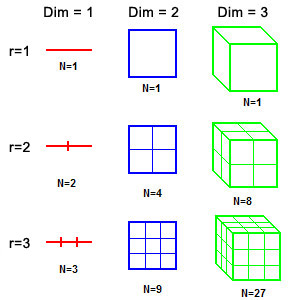# FractalsHasn’t symmetry always mesmerized you? Fractals are special objects that are symmetric with respect to scale.

### What are Fractals?

Fractals are beautiful mathematical patterns that are characterized by self-similarity. If a fractal pattern is zoomed into, the exact same pattern appears. Few popular examples are the Sierpinski Gasket and the Koch Snowflake.

### Fractal Dimensions

Fractals are also unique in that Fractal Dimension is not a whole number. As an example, the length of a Koch Curve is infinite while the area is zero. Hence, the dimension of the fractal is greater than one and less than two. Fractal dimensions can also be understood as a measure for space-filing capacity of a pattern.Let us now see an example on how to find the dimensions of a fractal: Koch Curve. Before that, consider a non-fractal object as shown and examine the pattern. If we magnify the line by a factor of r=2; its measure (length) increases to 2. If we do the same thing to a square and a cube, the measure increases by a factor of 4 and 8 respectively. Note that the relation N=r$^{D}$ holds, where N is the number of pieces (similar to r=1), r is the scaling factor and D is the dimension. Thus $D=\frac{log(N)}{log(r)}$

Now, let us try to apply similarly to Koch Curve:Scaling it by a factor of r=3, we get;There are N=4 pieces of the final object similar to the object or r=1 as shown. Hence, Dimension is $\frac{log(4)}{log(3)}=1.6$ Consider the Sierpinski GasketScale it by a factor of r=2We get N=3 pieces of the same size as that of r=1.Hence The Dimension is $\frac{log(3)}{log(2)}=1.58$

### Fractals in Nature

Although perfect Fractals cannot appear in nature because if you zoom further and further, we would eventually reach molecules and atoms (self-similarity stops at a much higher scale) which are not similar to the whole fractal; approximate fractals are found in nature having self-similarity over a finite range of scales. Brownian motion is an example of approximate fractal. Fractals are a very efficient design for plants to maximize their exposure to sun and cardiovascular systems to most efficiently transport oxygen to all parts of the body.The video below shows how fractals can appear in mathematical constructs.

Here is a Geogebra workspace where you can fiddle around with parameters to get a fractal.

### Mandelbrot Set

Mandelbrot was a French and American mathematician who referred to himself as the “fractalist”. He coined the word “Fractal” and is recognized for his contribution to fractal geometry. The Mandelbrot Set, one of the most famous fractals is the set obtained from the quadratic recurrence equation $z_{n+1}=z_n^2+C$ with $z_0=C$ ,where points C in the complex plane for which the orbit of zn does not tend to infinity are in the set.

### Why study fractals?

“Clouds are not spheres, mountains are not cones, coastlines are not circles, and bark is not smooth, nor does lightning travel in a straight line.” ― Benoît B. Mandelbrot

Study of Fractals has applications in a wide range of fields. Fractals are used to model natural objects like clouds, mountains, etc in Computer science. Fractals are used to create landscapes that are otherwise impossible with conventional technology in shows like Star Wars and Star trek. Fractals are commonly used in chaos theory as graphs of chaotic processes are fractals. Hilbert’s Space filling Curve and other such space-filing curves are fractals. It also finds application in Image Compressing. Certain algorithms are used to convert parts of images that have resemblance with other parts of the image into mathematical data called “fractal codes” which are used to recreate the encoded image.Here is a TED talk by Benoit Mandelbrot on” Fractals and the Art of Roughness”.